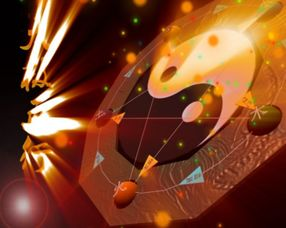# 五行水两画

2笔画的五行属水的字有：

3笔画的五行属水的字有：

4笔画的五行属水的字有：

5笔画的五行属水的字有：

## 5.五行里面属水的字有哪些

《五行属水》二划勹（水） 卜（水） 乜（水） 三划凡（水） 亡（水） 下（水） 子（水） 四划巴（水） 比（水） 不（水） 歹（水） 反（水） 方（水） 分（水） 夫（水） 父（水） 互（水） 户（水） 化（水） 幻（水） 毛（水） 爿（水） 匹（水） 片（水） 壬（水） 卅（水） 水（水） 文（水） 毋（水） 勿（水） 五划叭（水） 白（水） 半（水） 包（水） 北（水） 必（水） 弁（水） 卟（水） 布（水） 弗（水） 付（水） 夯（水） 禾（水） 弘（水） 乎（水） 矛（水） 民（水） 皿（水） 末（水） 母（水） 仫（水） 目（水） 丕（水） 皮（水） 氕（水） 平（水） 叵（水） 仨（水） 兄（水） 玄（水） 穴（水） 疋（水） 匝（水） 六划扒（水） 百（水） 冰（水） 并（水） 凼（水） 伐（水） 帆（水） 泛（水） 犯（水） 仿（水） 妃（水） 份（水） 缶（水） 伏（水） 亥（水） 好（水） 合（水） 冱（水） 回（水） 米（水） 糸（水） 名（水） 牟（水） 仳（水） 牝（水） 乒（水） 收（水） 汀（水） 危（水） 向（水） 刑（水） 行（水） 凶（水） 休（水） 血（水） 印（水） 汁（水） 七划吧（水） 夿（水） 弝（水） 伴（水） 贝（水） 伻（水） 皀（水） 佊（水） 吡（水） *（水） 庇（水） 别（水） 兵（水） 伯（水） 孛（水） 吥（水） 步（水） 汊（水） 池（水） 泛（水） 妨（水） 彷（水） 吠（水） 吩（水） 佛（水） 否（水） 呋（水） 孚（水） 甫（水） 汞（水） 佝（水） 含（水） 罕（水） 汗（水） 亨（水） 宏（水） 囫（水） 弧（水） 汲（水） 即（水） 江（水） 戒（水） 况（水） 冷（水） 忙（水） 尨（水） 每（水） 芈（水） 妙（水） 尿（水） 妞（水） 伾（水） 屁（水） 汝（水） 汕（水） 汜（水） 忘（水） 尾（水） 污（水） 汐（水） 希（水） 孝（水） 形（水） 汛（水） 妤（水） 妘（水） 八划岸（水） 八（水） 把（水） 爸（水） 扳（水） 攽（水） 版（水） 扮（水） 姅（水） 孢（水） 卑（水） *（水） 沘（水） 彼（水） 畀（水） 忭（水） 抃（水） 汳（水） 汴（水） 表（水） 秉（水） 幷（水） 帛（水） 瓝（水） 沉（水） 沌（水） 泛（水） 房（水） 放（水） 非（水） 氛（水） 汾（水） 忿（水） 奉（水） 扶（水） 府（水） 咐（水） 阜（水） 侅（水） 冈（水） 汩（水） 卦（水） 沆（水） 呵（水） 劾（水） 和（水） 佫（水） 呼（水） 虎（水） 或（水） 泐（水） 盲（水） 牦（水） 没（水） 妹（水） 门（水） 氓（水） 孟（水） 汨（水） 宓（水） 明（水） 命（水） 殁（水） 沫（水） 侔（水） 姆（水） 沐（水） 牧（水） 忸（水） 扭（水） 狃（水） 抛（水） 咆（水） 庖（水） 呸（水） 沛（水） 佩（水） 帔（水） 朋（水） 批（水） 沏（水） 汔（水） 汽（水） 沁（水） 沙（水） 沈（水） 汰（水） 汪（水） 味（水） 汶（水） 沃（水） 武（水） 物（水） 弦（水） 冼（水） 享（水） 协（水） 忻（水） 幸（水） 汹（水） 沂（水） 雨（水） 沅（水） 咂（水） 沚（水） 状（水） 九划疤（水） 拔（水） 拜（水） 拌（水） 保（水） 抱（水） 背（水） 祊（水） 甭（水） 泵（水） 毖（水） 扁（水） 窆（水） 拼（水） 便（水） 昪（水） 波（水） 泊（水） 勃（水） 哺（水） 怖（水） 匆（水） 沲（水） 法（水） 畈（水） 飞（水） 沸（水） 狒（水） 玢（水） 风（水） 封（水） 凫（水） 怫（水） 拂（水） 俘（水） 氟（水） 罘（水） 拊（水） 讣（水） 负（水） 泔（水） 沽（水） 哈（水） 孩（水） 河（水） 很（水） 红（水） 泓（水） 侯（水） 后（水） 厚（水） 狐（水） 怙（水） 徊（水） 奂（水） 宦（水） 皇（水） 虺（水） 哕（水） 计（水） 沮（水） 炬（水） 姥（水） 泪（水） 泠（水） 泖（水） 昴（水） 冒（水） 玫（水） 眉（水） 美（水） 昧（水） 虻（水） 咪（水） 弭（水） 泌（水） 沔（水） 勉（水） 眇（水） 秒（水） 咩（水） 抿（水） 泯（水） 抹（水） 哞（水） 某（水） 拇（水） 泥（水） 拍（水） 哌（水） 泮（水） 叛（水） 盼（水） 狍（水） 泡（水） 疱（水） 怦（水） 抨（水） 披（水） 毗（水） 姘（水） 品（水） 屏（水） 泼（水） 匍（水） 柒（水） 泣（水） 泅（水） 泉（水） 染（水） 娆（水） 沭（水） 泗（水） 沱（水） 咸（水） 香（水） 巷（水）。